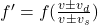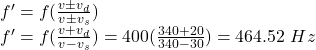## The frequency of the sound heard by the motorcyclist is given by the expression A motorcyclist moves to the left with a speed of 20 m/

Question

The frequency of the sound heard by the motorcyclist is given by the expression
A motorcyclist moves to the left with a speed of 20 m/s. A police car with velocity 30 m/s to the right emits a sound with a
frequency of 400 Hz. Speed of sound in air is 340 m/s.

in progress 0
5 months 2021-08-24T23:23:30+00:00 1 Answers 3 views 0

464.52 Hz

Explanation:

This is a Doppler effect, the Doppler effect causes a change in frequency when the source of the wave is moving or the listener is moving. The general equation is given by:Where f is the frequency of the source = 400 Hz, v is Speed of sound in air = 340 m/s, vd is the velocity of the observer = 20 m/s and vs is the velocity of the source = 30 m/s

Since the observer is moving towards the source, the numerator is v + vd. The source is also moving towards the observer therefore the denominator is v – vs.

The frequency of the sound heard by the motorcyclist (f’) is given by the expression: